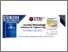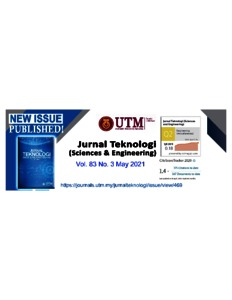# The simulation of mechanical stimulation effect on bone elasticity limit based on finite element method (FEM)

Yakin, Khusnul and Styaningsih, Ita and Rusmana, Nurani I and Tirono, Mohammad and Umam, Rofiqul (2021) The simulation of mechanical stimulation effect on bone elasticity limit based on finite element method (FEM). Jurnal Teknologi, 83 (3).Preview
Text
9049.pdf - Published Version

## Abstract

Osteoporosis is a disease affecting bones which is characterized by decreased bone density; bones become porous and susceptible to fractures. Osteoporosis
occurs because of an imbalance during bone remodeling phase between resorption and formation processes. This study aims to simulate the effects of mechanical stimulations on the femoral bone elasticity limit. It is hoped that
these mechanical stimuli can provide information on bone elasticity limits. Initially, we constructed the femur in two layers using triangular elements. Then
we entered the bone properties (Young’s modulus and Poisson’s ratio) based on the age of the femur. After that we calculated the value of the stress, strain, and
strain rate in the reversal phase. Next, we calculated the bone density using the thermodynamic equation and calculation of the bone elasticity limit using
particle swarm optimization (PSO) methods. The value of stress and strain caused by walking is higher than the value of stress and strain when standing still. In this
case, the difference in activity results an increase in stress by 33.82% and an increase in strain and strain rate by 34.57%. Based on these simulation results, it
can be concluded that mechanical stimulation can increase the limit of bone elasticity to 2.99% in cortical bone and 0.975% in trabecular bone. Bone elasticity
limit can be used to determine the level of osteoporosis that occurs. The higher value of the bone elasticity, the smaller the possibility of osteoporosis.

Item Type: Journal Article Bone remodelling, bone density, elasticity limit, finite element method, mechanical stimulation 02 PHYSICAL SCIENCES > 0299 Other Physical Sciences > 029901 Biological Physics02 PHYSICAL SCIENCES > 0299 Other Physical Sciences > 029903 Medical Physics01 MATHEMATICAL SCIENCES > 0102 Applied Mathematics01 MATHEMATICAL SCIENCES > 0103 Numerical and Computational mathematics01 MATHEMATICAL SCIENCES > 0105 Mathematical Physics Faculty of Mathematics and Sciences > Department of Physics Khusnul Yakin 08 Jul 2021 12:21View Item